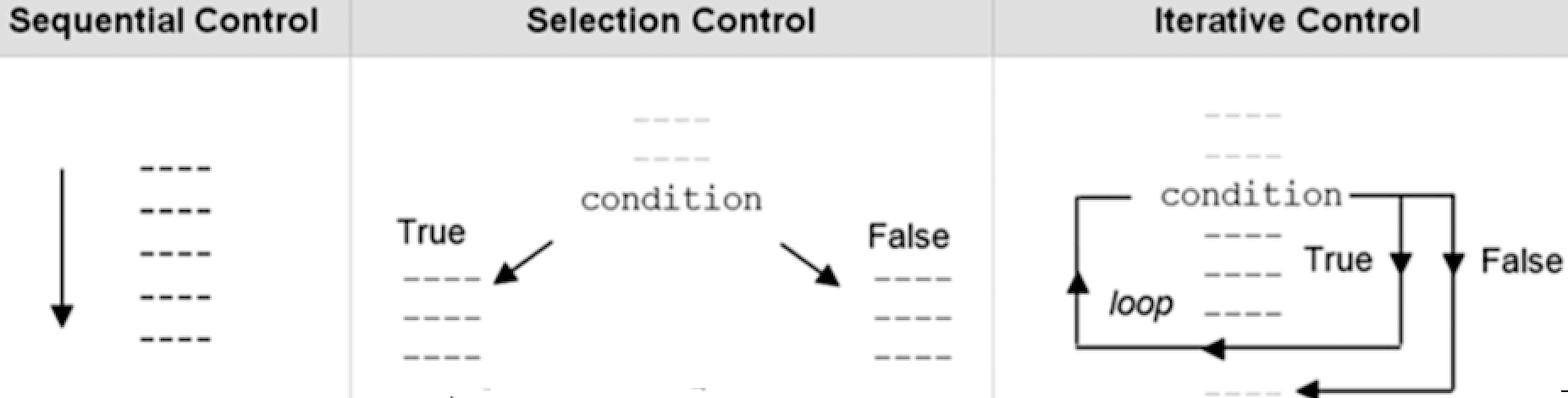•510week1

# 510week1

NOTE笔记

## 最佳编程实战

### 什么是程序

input输入，从键盘,麦克风,GPS等输出数据

process过程,通过CPU计算

out输出 将结果显示显示器,存储或播放

### 程序的基本构件

1.sequential 顺序(自上而下执行)

2.selective 选择性(决策执行)

3.Iteaative or "Loop repetition " 迭代或循环重复## java基础知识

• Variables 变量： 存储在内存中的值，可以更改，建立良好的命名习惯

​ Ex. emp_Rate or empRate empHours , empId

• Constants 常量: 存储在内存中且不能更改的值 使用关键字-> `final`

`final int ival = 20; //value needed immediately upon declaration!`

##基本数据类型

byteRandom
booleanString
floatfloat
doubledouble
intInteger
shortShort
longLong
charCharacter
``````int j=0, k=1;
double a = 2.0;
double b = 5;
``````

``````int result = 3/4;  //0 as truncation occurs
``````

### 优先&关联

Arithmetic 算术

Conditionals ( and && , or || , not ! ) 条件句 ( 和 && ，或 || ，不是 ! )

Relational ( > , < , >= , <= , != , == ) 关系( > ， < ， >= ， <= ， != ， == )

``````++count;  //pre increment operator (change to variable takes place immediately)
// vs.
count++;  //post increment operator (change to variable takes place after assignment ends)

// same as
count = count + 1;
// or
count+=1;
``````

``````int a = 12;

(5 == a)
``````

``````String name1 = "Joe", name2 = "Jack";

(name1.equals(name2))
``````

### 条件句

``````if(a>b)
//if true do something
else
//if false do something else
``````

``````if(a>b) {
result = a;
System.out.print("Value = " + result);
}
else {
result = b;
System.out.print("Value = " + result);
}
``````

### 其他形式

``````int result = a>b ? a : b;

// if statement equivalent

if(a>b)
result = a;
else
result = b;
``````### loops 循环

``````int count = 0;
while (count<10)  {
//do something
count++;  //increment counter variable
}
``````

Arrays 数组

``````int array[] = {1,2,3,4,5};

array=14; //update array at subscript 2, element #3
``````
Element number元素编号Subscript number下标编号Value at subscript position 下标位置的值
101
212
3214
434
545

Ex. Cycle thru array up to array length - 1 循环数组，最大数组长度为-1

``````for (int i = 0; i < array.length; i++)
System.out.print(array[i] + " ");
``````

## 单选

1. 解决问题过程的第一步是在编程语言 (如Java)中实现算法，并验证算法是否有效。

Define Problem 定义问题 Create (suggest) a solution (s) 创建一个解决方案 Code away!! 编写代码 Test 测试 Maintenance (ongoing) （持续）维护 维护(持续)(持续)维护

1. 符号 `'5'` 不属于字符数据类型，因为5是数字。❌

2. 如果在表达式中使用了 ++x ，则首先计算该表达式，然后将 x 的值递增 1 ❌ ++x指的是先加再运算.

3. 在Java中， `!``&&``||` 称为逻辑运算符。

Arithmetic 算数 算术算数 Conditional 条件 有条件的条件 Relational 关系 关系关系

1. 假设 P 和 Q 是逻辑表达式。如果 P 和 Q 都为假，则逻辑表达式 P && Q 为假

Ex. P => A>B , Q => A==2 is P&&Q a true outcome

• `Ex 假设所有变量都被正确声明，Java代码的输出是32。` ❌正确答案是35
``````num = 10;
while (num <= 32)
num = num + 5;
System.out.println(num);
``````

1. A constructor has no type and is therefore a void method.构造函数没有类型，因此是一个空方式❌

译：构造函数没有类型，因此是一个空方法。 PURPOSE: SET THE OBJECT(S) TO AN INITIAL STATE. 目的：将对象设置为初始状态。 构造函数没有 返回类型，所以没有 void

2. Given the declaration 鉴于这份声明

``````double\[\] numList = new double\[20\]; //0-19 INDICIE
``````

the statement 这份声明

``````numList\[12\] = numList\[5\] + numList\[7\];
``````

1. A subclass can override public methods of a superclass.子类可以重写超类的 `public` 个方法

2. If an exception occurs in a try block and that exception is caught by a catch block, then the remaining catch blocks associated with that try block are ignored.

## 多选

(答案1为正确,0为错误)

1. To develop a program to solve a problem, you start by .要开发解决问题的程序，您可以从 1000

analyzing the problem implementing the solution in Java designing the algorithm 0 entering the solution into a computer system\

• 分析问题

• 用 JAVA 实现解决方案 -

• 设计算法

• 将解决方案输入计算机

1. the first step in OOD is to identify the components called .面向对象设计的第一步是识别称为 的组件0100 classes

objects

methods

data

1. Which of the following is a valid int value? 以下哪一项是有效的整数值 1000

3279

3,279

3270.00

-922337203684547758808

1. Which of the following is a valid statement? 以下哪一项陈述是正确的？ 0010

(i) `int num = new int(67);` (ii) `String name = new ("Doe");` (iii) `String name = "Doe";`

• Only (i)
• Only (i) and (ii)
• Only (iii)
• Only (ii) and (iii)
1. Declaring a class level variable as static means .将类级别变量声明为静态表示为 0100

access is obtainable only thru a class object any method (static, instance) can have access no methods can have access none of the above

2. Consider the following statements

``````double x; String y; y = String.format("%.2f", x);
``````

``````    "285.00"
"285.680"
"285.68"
"285.068"
``````
1. What is the output of the following Java code? 0010
``````int x = 0;
if (x > 0)
System.out.println("positive ");
System.out.println("zero ");
System.out.println("negative");
``````

• zero
• negative
• zero negative
• positive zero negative
1. If str1 is "Hello" and str2 is "Hi", which of the following could be a result of `str1.compareTo(str2);` ? 0100

• 4
• -4
• -1
• 1
2. If str1 is "Hello" and str2 is "Hi", which of the following could be a result of `str2.compareTo(str1);` ? 1000

• 4
• -4
• -1
• 1
1. If str1 is "Hello" and str2 is "Hello", which of the following could be a result of str1.compareTo(str2); ? 如果str1为“Hello”，而str2为“Hello”，则以下哪一项可能是 str1.compareTo(str2); 的结果？ 0010 4 -4 0 1